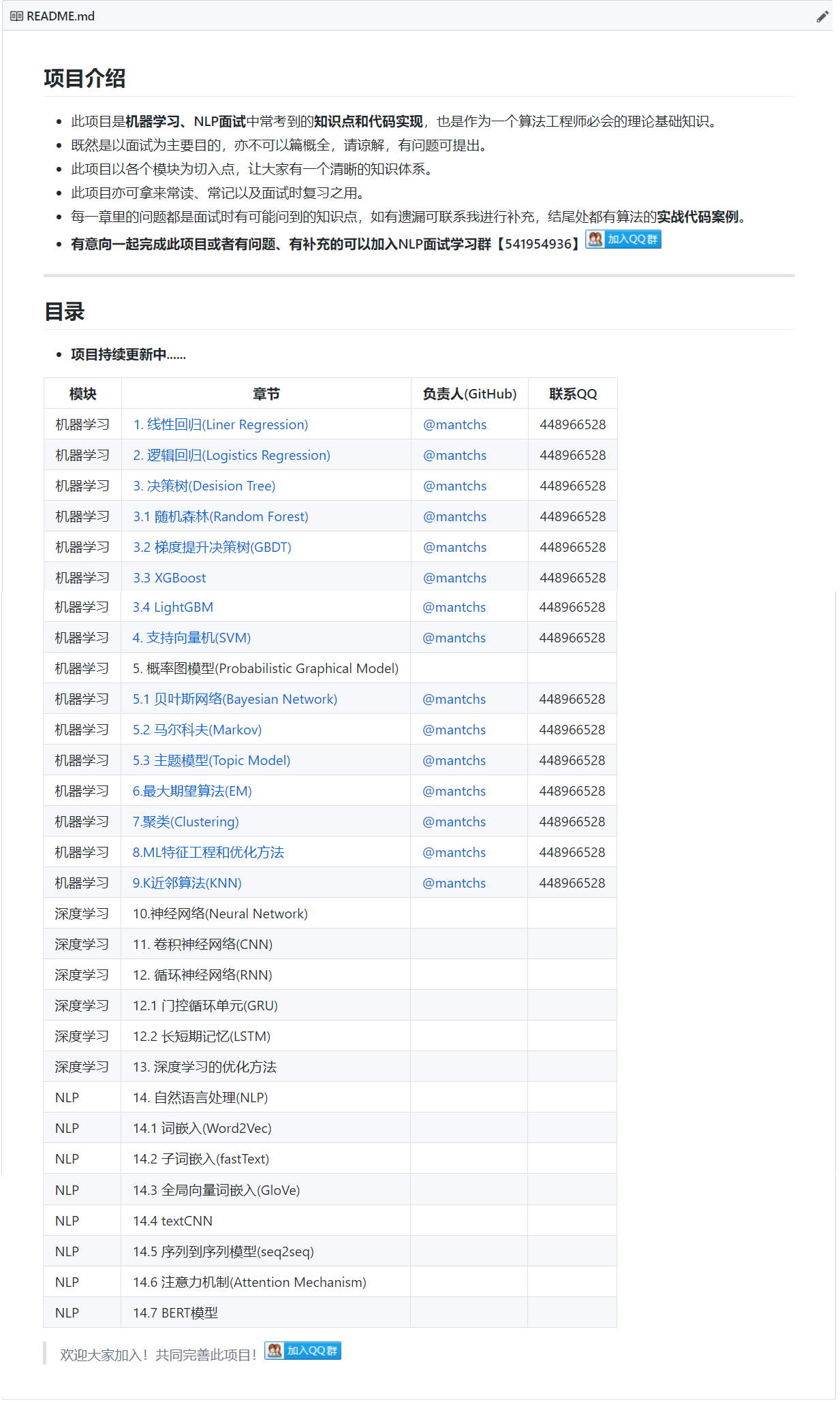# 【深度学习】门控循环神经⽹络(GRU)

## 2. ⻔控循环单元

### 2.1 重置门和更新门

GRU它引⼊了重置⻔（reset gate）和更新⻔（update gate）的概念，从而修改了循环神经⽹络中隐藏状态的计算⽅式。$$R_t=\sigma(X_tW_{xr}+H_{t-1}W_{hr}+b_r)$$

$$Z_t=\sigma(X_tW_{xz}+H_{t-1}W_{hz}+b_z)$$

sigma函数可以将元素的值变换到0和1之间。因此，重置⻔ $R_t$ 和更新⻔ $Z_t$ 中每个元素的值域都是[0, 1]。

### 2.2 候选隐藏状态$$\tilde{H}t=tanh(X_tW{xh}+(R_t⊙H_{t-1})W_{hh}+b_h)$$

### 2.3 隐藏状态• 重置⻔有助于捕捉时间序列⾥短期的依赖关系；
• 更新⻔有助于捕捉时间序列⾥⻓期的依赖关系。

## 3. 代码实现GRU

MNIST–GRU实现《动手学–深度学习》